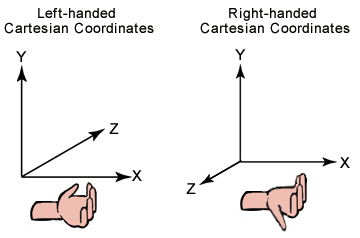# 左右手坐标系# 向量的模

//! Get length of the vector.
T getLength() const { return core::squareroot( X*X + Y*Y + Z*Z ); }

//! Get squared length of the vector.
/** This is useful because it is much faster than getLength().
/return Squared length of the vector. */
T getLengthSQ() const { return X*X + Y*Y + Z*Z; }


DirectX中的实现差不多一样, 只是使用的是C风格的接口没有使用C++的类而已.

D3DXINLINE FLOAT D3DXVec3Length( CONST D3DXVECTOR3 *pV )
{
#ifdef D3DX_DEBUG
if(!pV)
return 0.0f;
#endif

#ifdef __cplusplus
return sqrtf(pV->x * pV->x + pV->y * pV->y + pV->z * pV->z);
#else
return (FLOAT) sqrt(pV->x * pV->x + pV->y * pV->y + pV->z * pV->z);
#endif
}

D3DXINLINE FLOAT D3DXVec3LengthSq( CONST D3DXVECTOR3 *pV )
{
#ifdef D3DX_DEBUG
if(!pV)
return 0.0f;
#endif

return pV->x * pV->x + pV->y * pV->y + pV->z * pV->z;
}


MATLAB中用norm函数取模:

>> a = [1, 1, 1]

a =

1     1     1

>> b = norm(a)

b =

1.7321


# 三维空间中两点的距离

Irrlich的实现:

//! Get distance from another point.
/** Here, the vector is interpreted as point in 3 dimensional space. */
T getDistanceFrom(const vector3d<T>& other) const
{
return vector3d<T>(X - other.X, Y - other.Y, Z - other.Z).getLength();
}

//! Returns squared distance from another point.
/** Here, the vector is interpreted as point in 3 dimensional space. */
T getDistanceFromSQ(const vector3d<T>& other) const
{
return vector3d<T>(X - other.X, Y - other.Y, Z - other.Z).getLengthSQ();
}


# 向量的规范化

//! Normalizes the vector.
/** In case of the 0 vector the result is still 0, otherwise
the length of the vector will be 1.
/return Reference to this vector after normalization. */
vector3d<T>& normalize()
{
f64 length = (f32)(X*X + Y*Y + Z*Z);
if (core::equals(length, 0.0)) // this check isn't an optimization but prevents getting NAN in the sqrt.
return *this;
length = core::reciprocal_squareroot ( (f64) (X*X + Y*Y + Z*Z) );

X = (T)(X * length);
Y = (T)(Y * length);
Z = (T)(Z * length);
return *this;
}

 上述代码中首先计算length以防其为0, 然后直接计算$$\frac{1}{| u }$$, （这样做的目的从代码实现上来看是因为SSE,Nviadia都有可以直接计算此值的能力） 然后再分别与各坐标值进行乘法运算.

DirectX中的API：(无实现可看）

D3DXVECTOR3* WINAPI D3DXVec3Normalize ( D3DXVECTOR3 *pOut, CONST D3DXVECTOR3 *pV );


# 向量的加减法, 数乘

## 向量的加法

AB+BC=AC.

a+b=(x+x’, y+y’).

a+0=0+a=a.

## 向量的减法

AB-AC=CB. 即“共同起点, 指向被减”

a=(x,y) b=(x’,y’) 则 a-b=(x-x’,y-y’).

# 点积(dot product)又称数量积或内积

 点积等于向量v0的长度乘以v1的长度, 再乘以它们之间夹角的余弦, 即 v0 * v1 *cos(θ).

 cos(θ)=v0.v1/ v0 v1 ;
 θ=Math.acos(v0.v1/ v0 v1 );

θ=Math.acos(v0.v1);

V0.v1=0 =》两个向量互相垂直

V0.v1>0 =》两个向量的夹角小于90度

V0.v1<0 =》两个向量的夹角大于90度

Irrlicht中的实现: (很简单的公式, 很直白的实现)

//! Get the dot product with another vector.
T dotProduct(const vector3d<T>& other) const
{
return X*other.X + Y*other.Y + Z*other.Z;
}


DirectX中的实现：（很简单的公式, 也是很直白的实现）

D3DXINLINE FLOAT D3DXVec3Dot( CONST D3DXVECTOR3 *pV1, CONST D3DXVECTOR3 *pV2 )
{
#ifdef D3DX_DEBUG
if(!pV1 || !pV2)
return 0.0f;
#endif

return pV1->x * pV2->x + pV1->y * pV2->y + pV1->z * pV2->z;
}


# 叉积(cross product): 也称向量积

Irrlicht的实现:

//! Calculates the cross product with another vector.
/** /param p Vector to multiply with.
/return Crossproduct of this vector with p. */
vector3d<T> crossProduct(const vector3d<T>& p) const
{
return vector3d<T>(Y * p.Z - Z * p.Y, Z * p.X - X * p.Z, X * p.Y - Y * p.X);
}


DirectX的实现:

D3DXINLINE D3DXVECTOR3* D3DXVec3Cross
( D3DXVECTOR3 *pOut, CONST D3DXVECTOR3 *pV1, CONST D3DXVECTOR3 *pV2 )
{
D3DXVECTOR3 v;

#ifdef D3DX_DEBUG
if(!pOut || !pV1 || !pV2)
return NULL;
#endif

v.x = pV1->y * pV2->z - pV1->z * pV2->y;
v.y = pV1->z * pV2->x - pV1->x * pV2->z;
v.z = pV1->x * pV2->y - pV1->y * pV2->x;

*pOut = v;
return pOut;
}


MATLAB中叉积用的是cross函数:

>> a = [2,2,1]

a =

2     2     1

>> b= [4,5,3]

b =

4     5     3

>> c = cross(a,b)

c =

1    -2     2


Irrlicht版本:

#include <stdio.h>
#include <irrlicht.h>
using namespace irr::core;

int _tmain(int argc, _TCHAR* argv[])
{
vector3df a(2.0f, 2.0f, 1.0f);
vector3df b(4.0f, 5.0f, 3.0f);

vector3df c = a.crossProduct(b);

printf("c = (%f, %f, %f)", c.X, c.Y, c.Z);

return 0;
}


c = (1.000000, -2.000000, 2.000000)

DirectX版本:

#include <stdio.h>
#include <d3dx9.h>

int _tmain(int argc, _TCHAR* argv[])
{
D3DXVECTOR3 a(2.0f, 2.0f, 1.0f);
D3DXVECTOR3 b(4.0f, 5.0f, 3.0f);

D3DXVECTOR3 c;
D3DXVec3Cross(&c, &a, &b);

printf("c = (%f, %f, %f)", c.x, c.y, c.z);

return 0;
}



c = (1.000000, -2.000000, 2.000000)

# 参考资料：

1. DirectX 9.0 3D游戏开发编程基础, （美）Frank D.Luna著, 段菲译, 清华大学出版社
2. 大学数学 湖南大学数学与计量经济学院组编, 高等教育出版社
3. 百度百科及wikipedia

# 修改记录

2015年10月, 将原来用Freemat及Ocatave实现的部分, 改为MATLAB, 原文使用Live Writer编写, 新改为Markdown格式.

Posted By 九天雁翎 at 九天雁翎的博客 on 2009年12月08日